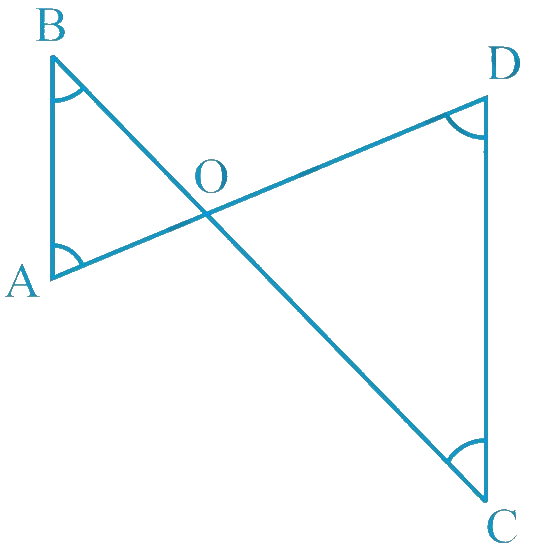In the verge of coronavirus pandemic, we are providing FREE access to our entire Online Curriculum to ensure Learning Doesn't STOP!

# Ex.7.4 Q3 Triangles Solution - NCERT Maths Class 9

Go back to  'Ex.7.4'

## Question

In the given figure, $$\angle B \lt \angle A$$ and

$$\angle C \lt \angle D$$. Show that $$AD \lt BC$$.

Video Solution
Triangles
Ex 7.4 | Question 3

## Text Solution

What is Known?

$\angle \text{B}<\angle \text{A and }\angle \text{C}<\angle \text{D}$

To prove:

$\text{AD}<\text{BC}$

Reasoning:

We can use the fact that In any triangle, the side opposite to the larger (greater) angle is longer We can add both the triangles result to get the required result.Steps:

In $$\Delta AOB$$,

\begin{align}&\angle B \lt \angle A\\ &AO \lt BO\\& \left(\begin{array}{}\text{Side opposite to the smaller}\\ \text{ angle is smaller} \ldots (1)\end{array}\right)\end{align}

In $$\Delta COD$$,

\begin{align}&\angle C \lt \angle D\\ &OD \lt OC\\& \left(\begin{array}{}\text{Side opposite to the smaller}\\ \text{ angle is smaller} \ldots (2)\end{array}\right)\end{align}

On adding Equations ($$1$$) and ($$2$$), we obtain

\begin{align} & AO + OD \lt BO + OC \\ & AD \lt BC, \text{proved}\end{align}

Video Solution
Triangles
Ex 7.4 | Question 3

Learn from the best math teachers and top your exams

• Live one on one classroom and doubt clearing
• Practice worksheets in and after class for conceptual clarity
• Personalized curriculum to keep up with school